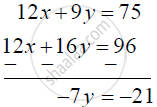SSC (Marathi Semi-English) 10thMaharashtra State Board
Share

# Find the Value of X- Y If 4x + 3y = 25, 3x + 4y = 24 - SSC (Marathi Semi-English) 10th - Algebra

ConceptLinear Equations in Two Variables Applications

#### Question

Find the value of x- y  if 4x + 3y = 25, 3x + 4y = 24

#### Solution

4x + 3y = 25 .....(i)

3x + 4y = 24 .....(ii)

Equation (i) is multiply by 3 and (ii) by 4∴ y = 3

∴ 4x + 3(3) = 25

4x = 16

x = 4

∴ x - y = 4  -3 = 1

Is there an error in this question or solution?

#### APPEARS IN

Solution Find the Value of X- Y If 4x + 3y = 25, 3x + 4y = 24 Concept: Linear Equations in Two Variables Applications.
S